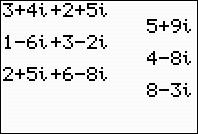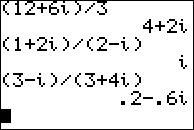# Activities

••• ##### Subject Area

• Math: Algebra II: Complex Numbers

• ##### Author9-12

15 Minutes

• ##### Device
• TI-83 Plus Family
• TI-84 Plus
• TI-84 Plus Silver Edition
•TI-84 Plus C Silver Edition
•TI-84 Plus CE

## Complex Numbers#### Activity Overview

Students calculate problems to determine the rules for adding, subtracting, multiplying, and dividing complex numbers.

#### Key Steps

•Students look for patterns to determine how the calculator adds, subtracts, multiplies and divides complex numbers. In each problem, students examine completed examples and discuss a process with a partner, then complete additional problems to confirm or refine the process.

•At the end of this activity, students will be able to discuss how to perform operations with complex numbers.